A client recently asked if there's a programmatic way to reduce memory requirements of a CAS table. In this post, you'll learn how to accomplish that when your SASWORK data set has lengthy character data.

## What is SASWORK?

The SASWORK library is the temporary library automatically defined by SAS at the start of each SAS session or job. The SASWORK library stores temporary SAS files that you create, such as data sets, catalogs, formats, etc., as well as files created internally by SAS. To access files in the SASWORK library, specify a one-level name for the file or by using a two-level name, i.e. the WORK libref.

## A programmatic CASLIB to SASWORK

It is a common practice for SAS programmers to read a source table and then store that source table in SASWORK. When the SASWORK data set has long character data, you can significantly reduce memory requirements by creating a path-based CASLIB to SASWORK and leveraging PROC CASUTIL with the CASDATA= option and use the IMPORTOPTIONS VARCHARCONVERSION=16 statement. The VARCHARCONVERSION=16 statement automatically converts all character data types with length of 16 or greater to a VARCHAR data type.

Code in Figure 1 below creates a macro variable called WORKPATH which we will use in our CASLIB statement:

```/* The macro variable WORKPATH contains the path to SASWORK */ %let workpath = %sysfunc(quote(%sysfunc(pathname(work)))); %put &workpath;```

(Figure 1: Creating the Macro Variable WORKPATH)

Figure 2 below is the resulting SAS log: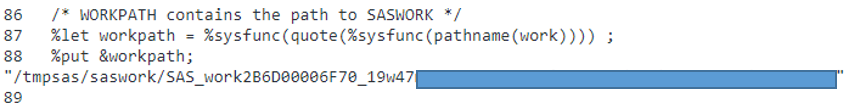(Figure 2: SAS log of creating the Macro Variable WORKPATH)

Code in Figure 3 below creates our SASWORK data set that we will lift into CAS:

```data saswork_table_with_char300; length a \$ 300 b \$ 15 c \$ 16; a='a300'; b='b15' ; c='c16' ; output; a='a300300'; b='b151515'; c='c161616'; output; c='c161616161616161'; b='b15151515151515'; a="a300qzwsxedcrfvtgbyhnujmiklopqazwsxedcrfvtgbyhnujmikolp1234567890123456789012345678901234567890"; output; run;   proc contents data=saswork_table_with_char300; title "Contents of WORK.SASWORK_TABLE_WITH_CHAR300"; run;```

(Figure 3: Code to create a SASWORK data set using a one-level name, i.e. saswork_table_with_char300)

Notice in Figure 4 below the lengths of the character variables a, b, and c: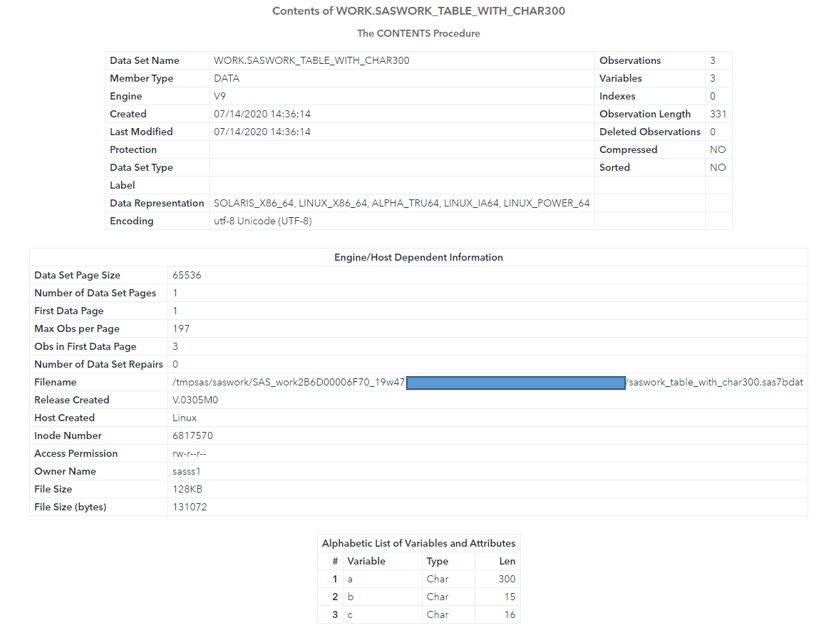(Figure 4: Results from PROC CONTENTS)

Code in Figure 5 below creates a path-based CASLIB to SASWORK by using our macro variable WORKPATH:

```proc cas; file log; table.dropCaslib / caslib='sas7bdat' quiet = true; run; addcaslib / datasource={srctype="path"} name="sas7bdat" path=&workpath ; run;```

(Figure 5: Path-based CASLIB to SASWORK)

Figure 6 below is resulting SAS log: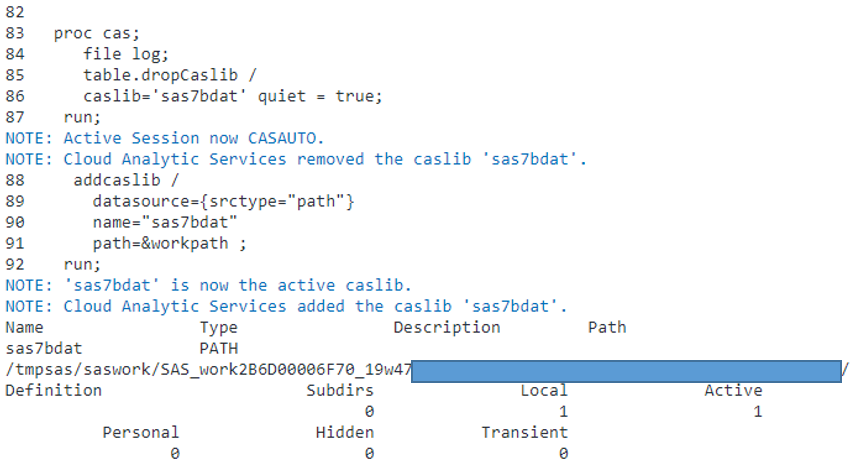(Figure 6: SAS log of path-based CASLIB to SASWORK)

Code in Figure 7 below lifts the SASWORK data set into CAS and ensures the CAS table will contain VARCHAR data types for any character data with a length of 16 or greater:

```proc casutil; load casdata="saswork_table_with_char300.sas7bdat" casout="cas_table_with_varchar" outcaslib="casuser" importoptions=(filetype="basesas", dtm="auto", debug="dmsglvli", varcharconversion=16) ; run; quit;```

(Figure 7: code that lifts the SASWORK data set into CAS)

Figure 8 below is the resulting SAS log: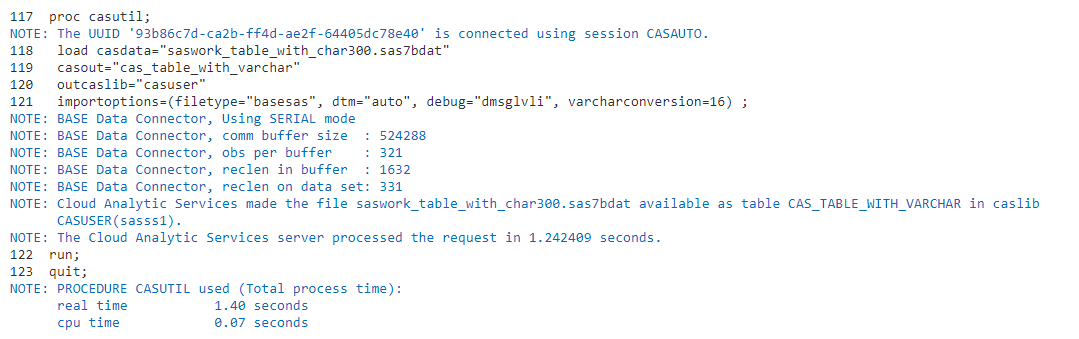(Figure 8: SAS log of code to lift the SASWORK data set into CAS)

Code in Figure 9 below displays the characteristics of our CAS table “cas_table_with_varchar”: Notice in Figure 10 that our variables a and c have a data type of VARCHAR.

```proc cas; sessionProp.setSessOpt / caslib="casuser"; run; table.columninfo / table="cas_table_with_varchar"; quit;```

(Figure 9: SAS code to display information of a CAS table)

Figure 10 below reveals CAS table characteristics: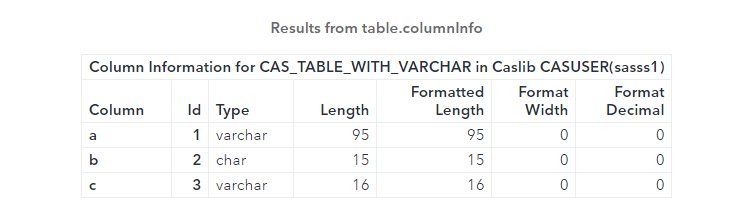(Figure 10: CAS table characteristics)

## Conclusion: Conserve memory with smaller CAS tables

By leveraging the coding techniques in this blog, we can lift into CAS any data sets we previously stored in SASWORK. In addition, we convert all character data types with a length of 16 or greater into the VARCHAR data type, which can significantly reduce the size of our CAS tables.

LEARN MORE | SAS® Cloud Analytic Services 3.5: User’s Guide